# Mathematics - Signals and Systems - Exercises on Linear Feedback Systems

in StemSocial2 months ago[Image1]

## Introduction

Hey it's a me again @drifter1!

Today we continue with my mathematics series about Signals and Systems to get into Exercises on Linear Feedback Systems.

So, without further ado, let's dive straight into it!

## Linear Feedback System Response Examples (Based on 25.4 from Ref1)

Consider simple feedback systems in continuous- and discrete-time respectively, as shown in the figure below.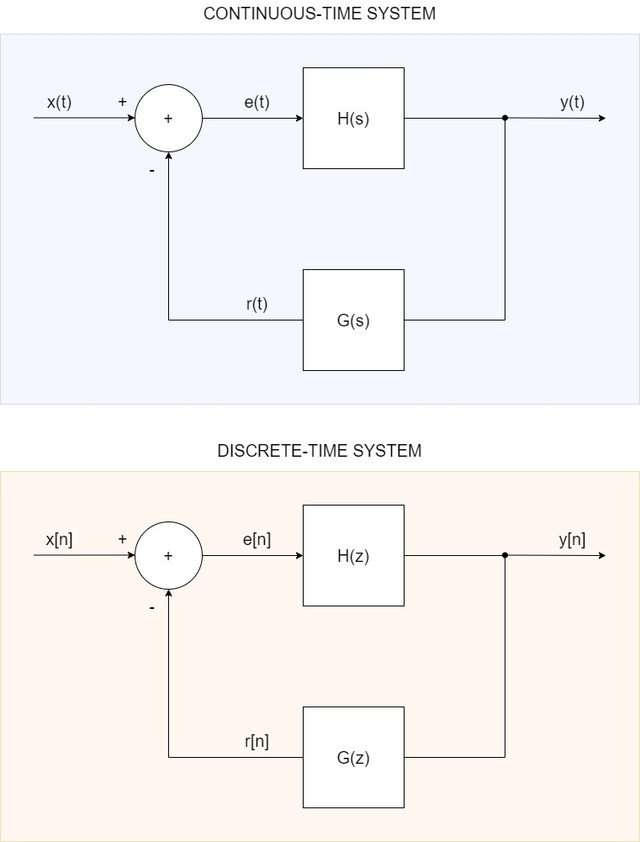Let's determine the closed loop system impulse response, v(t), for the continuous-time system, and system function, V(z), for the discrete-time system, in each of the following cases: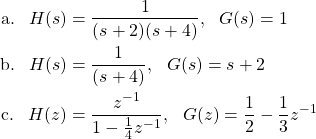### Solution

For both systems, the closed loop system function can be defined as: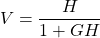#### a.

For the first case, the system function is: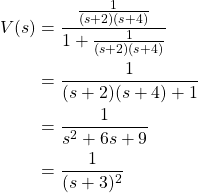Thus, from the Laplace Transform tables: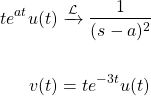#### b.

In the second case, we have: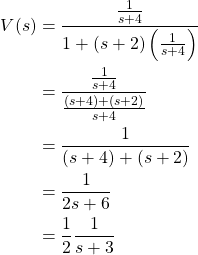Which, using the Laplace Transform tables and linearity property, yields the impulse response: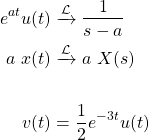#### c.

The third case and final case is for the discrete-time system. The closed loop system function is as follows: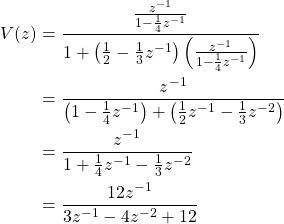```In the Z-Transform the calculations tend to become quite tedious,
and coming up with examples that simplify is also quite difficult.
So, let's just leave it there...```

## Linear Feedback System Stability Example (Based on 25.6 from Ref1)

Let's consider the causal discrete-time shown below.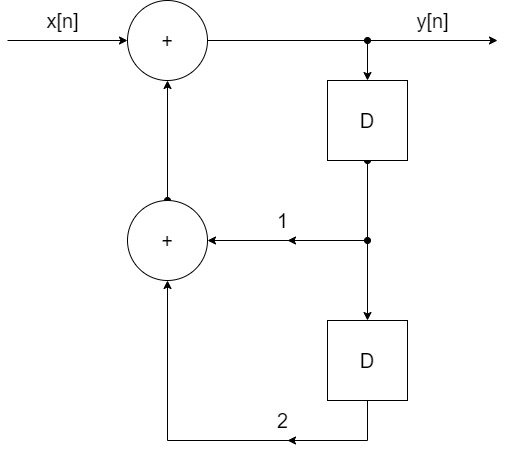• a. Proof that the system is not stable
• b. Suppose that there's feedback with one unit of delay, which means that the input is now given by: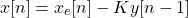where xe[n] is now the input to the overall system.
Determine the range of values of K (if such values exist) for which the system is stable.

### Solution

#### a.

In order to prove that the system is not stable, we have to proof that at least one pole is at |z| > 1. So, let's first find the system function that describes the system.

As a difference equation the system can be written as: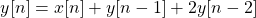Taking the Z Transform of the equation yields: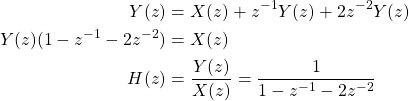The solutions of the denominator are the poles of the system. So, the poles of the system are located at: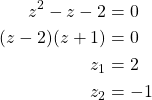Since |z1| = 2 > 1, the system is unstable.

#### b.

Introducing feedback of one unit of delay, the difference equation changes into: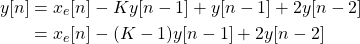Taking the Z Transform yields the system function: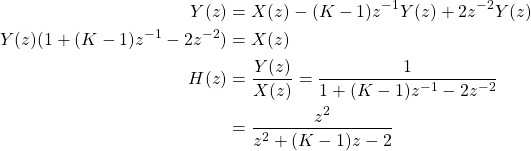The poles are now located at: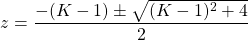For z = 1 we can find the range of K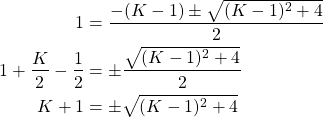The root term inside the square root is always positive, thus the roots are purely real. So, squaring both sides of the equation gives us the solution(s):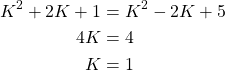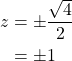So, the |z| < 1 condition is not satisfied. Thus, the system is not stable.

`You could keep going with two, three etc. units of delays as homework, until the system becomes stable!`

## RESOURCES:

### Images

Block diagrams and other visualizations were made using draw.io

## Previous articles of the series

### Laplace and Z Transforms

• Laplace Transform → Laplace Transform, Region of Convergence (ROC)
• Laplace Transform Properties → Linearity, Time- and Frequency-Shifting, Time-Scaling, Complex Conjugation, Multiplication and Convolution, Differentation in Time- and Frequency-Domain, Integration in Time-Domain, Initial and Final Value Theorems
• LTI System Analysis using Laplace Transform → System Properties (Causality, Stability) and ROC, LCCDE Representation and Laplace Transform, First-Order and Second-Order System Analysis
• Exercises on the Laplace Transform → Laplace Transform and ROC Examples, LTI System Analysis Example
• Z Transform → Z Transform, Region of Convergence (ROC), Inverse Z Transform
• Z Transform Properties → Linearity, Time-Shifting, Time-Scaling, Time-Reversal, z-Domain Scaling, Conjugation, Convolution, Differentation in the z-Domain, Initial and Final value Theorems
• LTI System Analysis using Z Transform → System Properties (Causality, Stability), LCCDE Representation and Z Transform
• Exercises on the Z Transform → Z Transform and ROC Examples, ROC from Conditions, LTI System Analysis Example
• Continuous-Time to Discrete-Time Design Mapping → Discrete-Time System Design Techniques (Mapping from Derivatives to Differences, Mapping using Impulse Invariance), First- and Second-Order Systems and Z-Transform
• Butterworth Filters → Butterworth Filter Parameters, Equation and Pole-Zero Plot, Mapping using Impulse-Invariance and Bilinear Transformation
• Linear Feedback Systems → System Feedback, Linear Feedback System Analysis

## Final words | Next up

This might actually be the end of the series, I'm not sure if there's much more to cover...

Also, this kind of posting has become quite boring and repetitive, so I'm looking for ways to spice things up. Maybe more coding, maybe more actual examples and exercises in these types of subjects (which are more on the theoretical side of things)...we shall see! But, you can be certain that the diversity of content will be enhanced thoroughly, because of the introduction of videos and maybe even streaming.

No more Mr. Text-guy!Keep on drifting!